# SSC Reasoning Ability Practice Questions (Day-62)

Dear Aspirants, The SSC Exams are going on at national level. Millions of candidates have eagerly prepared for the ongoing SSC exams such as CGL, CHSL, MTS, CPO, JE etc. SSC exams are usually conducted by the Staff Selection Commission to recruit the eligible candidates for various Central government departments. Mostly the selection process for all the SSC exams consists of the single or double phase of written examination based on the examination. Due to that, the written exam consisted of a certain syllabus and exam pattern. By following those regulations, the written exam has been conducted through an online mode. Based on the latest updated exam pattern and syllabus, here we have drafted the Reasoning Ability questions on a daily basis. So aspirants start your practice session with us and mould your skill in a perfect shape.

Start Quiz

Direction (1): In the following question, select the word which cannot be formed using the letters of the given word.

1)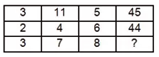(a) 72

(b) 76

(c) 80

(d) 84

2) How many triangles are there in the given figure?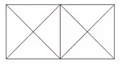(a) 16

(b) 18

(c) 20

(d) 22

3) How many quadrilaterals are there in the given figure?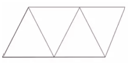(a) 5

(b) 6

(c) 8

(d) 10

Direction (4-5): In each of the following question below are given some statements followed by some conclusions. Taking the given statements to be true even if they seem to be at variance from commonly known facts, read all the conclusions and then decide which of the given conclusion logically follows the given statements.

4) Statements:

I) All men are scholars.

Conclusions:

I) All scholars are men.

(a) Only conclusion (I) follows

(b) Only conclusion (II) follows

(c) Only conclusion (III) follows

(d) No conclusion follows

5) Statements:

I) Some men are hardworking.

II) No blue is a advocate.

III) Some blue are men.

Conclusions:

I) Some blue are hardworking.

III) Some blue are not hardworking.

IV) Some men are not advocate.

(a) Only conclusion (IV)  and either (I) or (III) follows

(b) Only conclusion (II) and (IV) follow

(c) Only conclusion (I), (II) and (IV) follow

(d) Only conclusion (III) follows

Direction (6-7): From the given options, which answer figure can be formed by folding the figure given in the question?

6)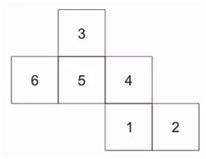(a)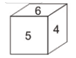(b)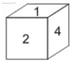(c)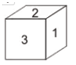(d)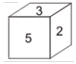7)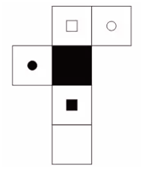(a)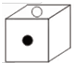(b)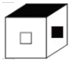(c)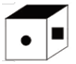(d)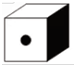8) In the given figure, which letter represents electronic mixer which is white?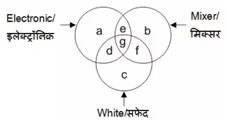(a) f

(b) g

(c) e

(d) b

9) In the given figure, which number represents yellow capsicum which is not rotten?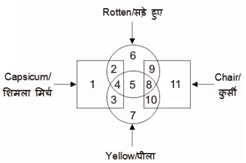(a) 4

(b) 3

(c) 2

(d) 5

10) In the given figure, what is the total of strong blades and sharp steel?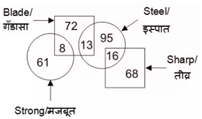(a) 24

(b) 16

(c) 29

(d) 69

32 + 11 + 52 = 45

22 + 4 + 62 = 44

32 + 7 + 64 = 80

18 triangles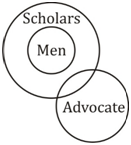I) ×

II) ×

III) ×

No conclusion follows.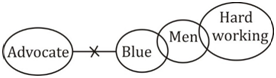Only IV and either I or III follows.

Opposite faces are

6 → 4

3 ↔ 1

5 ↔ 2

∴ Option (b) can be formed.

Opposite faces are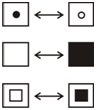Option (c) can be formed.

‘g’ represents electronic mixer which is white.

3 represents yellow capsicum which is not rotten.